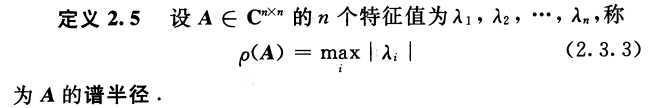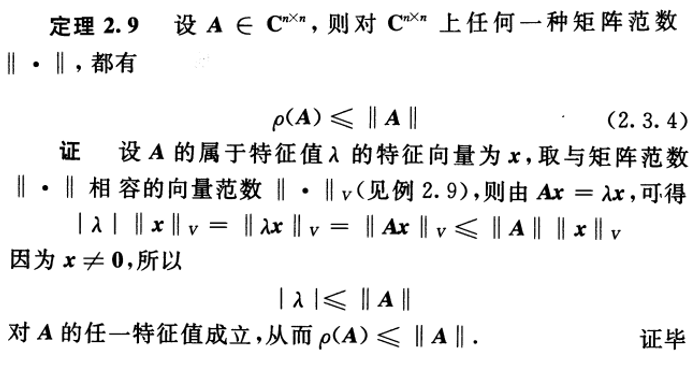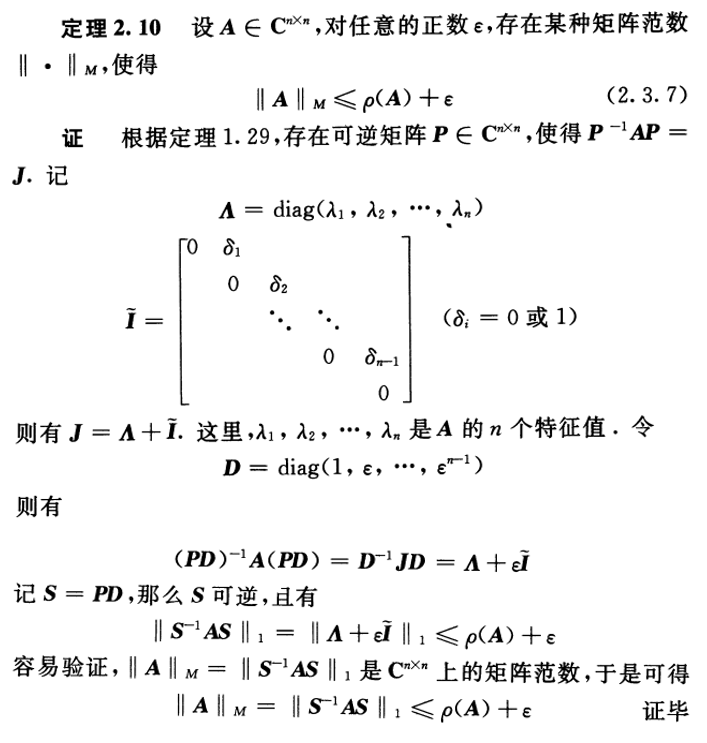• 矩阵的谱半径
万次阅读
2018-11-06 17:40:12
更多相关内容
• 矩阵谱半径指的是矩阵的最大特征值(含绝对值)。它可以判断收敛性，也可以判断方程解的稳定性。一般情况下，当存在一个单位矩阵减去另外一个矩阵的形式时， 谱半径小于一就是为了确保它们之间的差值为正这样逆矩阵才...

矩阵谱半径指的是矩阵的最大特征值(含绝对值)。

它可以判断收敛性，也可以判断方程解的稳定性。

一般情况下，当存在一个单位矩阵减去另外一个矩阵的形式时， 谱半径小于一就是为了确保它们之间的差值为正这样逆矩阵才会存在，可以用来验证一个方案是否可行。

The radius  of the smallest closed disc in the plane that contains the spectrum of this element (cf. Spectrum of an element). The spectral radius of an element  is connected with the norms of its powers by the formula

which, in particular, implies that  . The spectral radius of a bounded linear operator on a Banach space is the spectral radius of it regarded as an element of the Banach algebra of all operators. In a Hilbert space, the spectral radius of an operator is equal to the greatest lower bound of the norms of the operators similar to it (see ):

If the operator is normal, then  (cf. Normal operator).

定义：

Let λ1, ..., λn be the (real or complex) eigenvalues of a matrix A ∈ Cn × n. Then its spectral radius ρ(A) is defined as:

The following lemma shows a simple yet useful upper bound for the spectral radius of a matrix:

性质1.

Lemma: Let A ∈ Cn × n be a complex-valued matrix, ρ(A) its spectral radius and ||·|| aconsistent matrix norm; then, for each k ∈ N:

Proof: Let (v, λ) be an eigenvector-eigenvalue pair for a matrix A. By the sub-multiplicative property of the matrix norm, we get:

and since v ≠ 0 for each λ we have

and therefore

The spectral radius is closely related to the behaviour of the convergence of the power sequence of a matrix; namely, the following theorem holds:

2.

Theorem: Let A ∈ Cn × n be a complex-valued matrix and ρ(A) its spectral radius; then

if and only if

Moreover, if ρ(A)>1,  is not bounded for increasing k values.

From the Jordan normal form theorem, we know that for any complex valued matrix  , a non-singular matrix  and a block-diagonal matrix  exist such that:

with

where

It is easy to see that

and, since  is block-diagonal,

Now, a standard result on the  -power of an  Jordan block states that, for  :

Thus, if  then  , so that

which implies

Therefore,

On the other side, if  , there is at least one element in  which doesn't remain bounded as k increases, so proving the second part of the statement

3

For any matrix norm ||·||, we have

In other words, Gelfand's formula shows how the spectral radius of A gives the asymptotic growth rate of the norm of Ak:

for

谱半径与范数的关系：

1.定义：A是n阶方阵，λi是其特征值，i=1,2,…,n。则称特征值的绝对值的最大值为A的谱半径，记为ρ(A)。　注意要将谱半径与谱范数(2-范数)区别开来，谱范数是指A的最大奇异值，即A^H*A最大特征值的算术平方根。谱半径是矩阵的函数，但不是矩阵范数。

2、谱半径和范数的关系是以下几个结论：

定理1：谱半径不大于矩阵范数，即ρ(A)≤║A║。

因为任一特征对λ,x,Ax=λx，可得Ax=λx。两边取范数并利用相容性即得结果。

定理2：对于任何方阵A以及任意正数e，存在一种矩阵范数使得║A║

定理3(Gelfand定理)：ρ(A)=lim_{k->∞} ║A^k║^{1/k}。

利用上述性质可以推出以下两个常用的推论：

推论1：矩阵序列 I,A,A^2,…A^k,… 收敛于零的充要条件是ρ(A)<1。

推论2：级数 I+A+A^2+... 收敛到(I-A)^{-1}的充要条件是ρ(A)<1。

展开全文• 利用矩阵的有向图及有向图的1-path覆盖，给出非负矩阵谱半径与M矩阵最小特征值上下界的若干新估计，改进了已有的相应结果。
• 设计一种计算正矩阵谱半径及其特征向量的新算法,并证明算法的收敛性。结果表明,算法具有计算量小,便于实现,且能较快达到所需精度的特点。数值试验进一步验证了其可行性。
• 矩阵谱半径与范数的关系摘自 程云鹏. 矩阵论(第二版)[M]// 矩阵论（第二版）. 西北工业大学出版社, 2000. p135~p137

展开全文• 对非负矩阵谱半径的界值给出了一个新的估计,把非负矩阵谱半径的上下界表示成矩阵元素的一个易于计算的函数,证明了由该函数表示的谱半径的上下界可以通过递推计算的方法无限地逼近谱半径.最后,通过实例与以往的结论作...
• 设A=( ai,j) n×n为非负不可约矩阵,设计一种计算非负不可约矩阵谱半径ρ(A)的通用迭代算法,并证明算法的收敛性。数值实验表明,该算法比幂法迭代算法具有较快的收敛速度。
• 本文将改进的Gauss-Seidel迭代法应用于一类有很强应用背景的矩阵―H-矩阵及其比较矩阵，在较目前参考文献更一般的分裂条件下，得到相应的收敛结果及谱半径的比较结果，进而比较了其收敛速度的大小。所用方法不同于...
• 用矩阵的对角相似变换和 Perron-Frobenius定理,给出了不可约非负矩阵谱半径的简单数值算法,该算法类似于求矩阵按模最大特征值的经典算法幂法,适用于任何不可约非负矩阵,并且通过适当选择参数,算法具有简单、快速的...
• 借助2个新的矩阵,利用Frobenius G不等式,得出一种易于计算的新的估计方法,得出非负矩阵谱半径的上下界,最后通过实例说明该方法的优越性。
• 近四十年来许多文章致力于研究在系数矩阵是M-矩阵的情形下，线性方程组的预处理子的修改与完善，目的是为了改善古典迭代...从而得到在这种改进的预处理方法下，Jacobi及Gauss-Seidel迭代法的迭代矩阵谱半径的比较结果。
• 借助两个新的矩阵得到非负矩阵谱半径的两个新估计及其证明，并通过实例与以往的结论作比较，验证了这些估计的有效性及精确度。
• 在M是α-严格对角占优矩阵下估计迭代矩阵M-1N谱半径上界．通过计算|λ(M-1N)|需满足的条件得出了p(A-1)，P(J)的估计，并用数值算例说明了这些结论的有效性．
• 研究Pascal矩阵谱半径及其对应特征向量的数值求解算法问题,利用幂法和Pascal矩阵的性质给出了一个有效的迭代求解算法,该算法每一步迭代只用到浮点数的加法运算。同时数值实验显示,该算法具有较高的精度和较快的收敛...
• 概述：本道作业题是应镜拇同学的课后练习，分享的知识点是谱半径，指导老师为鲁老师，涉及到的知识点...矩阵A的特征值为λ1,λ2,……,λn,谱半径ρ(A)=max〔λi〕(i=1,2,……,n)相关例题题1：【如何证明矩阵谱半径...

概述：本道作业题是应镜拇同学的课后练习，分享的知识点是谱半径，指导老师为鲁老师，涉及到的知识点涵盖：【什么是矩阵的谱半径？怎么求？】百度-谱半径，下面是应镜拇作业题的详细。

题目：【什么是矩阵的谱半径？怎么求？】百度-谱半径

矩阵的谱半径就是指矩阵的特征值中绝对值最大的那个.

矩阵A的特征值为λ1,λ2,……,λn,谱半径ρ(A)=max〔λi〕(i=1,2,……,n)

相关例题

题1：

【如何证明矩阵谱半径不是矩阵范数】

证明：

记λ为矩阵A的模最大特征值(谱半径),x为其对应的右特征向量,那么：

x\'A\' × Ax = |λ|#178; × x\'x => |λ| = ||Ax||#8322;/ ||x||#8322;

题2：

【请问如何证明,矩阵的任何范数都不小于它的谱半径?】[数学]

必须是相容范数

证明很容易,取一个模最大的特征值及相应的特征向量:Ax=λx

然后 ρ(A)||x|| = ||λx|| = ||Ax||

题3：

一个矩阵,每一行元素的和都是定值(设为a),请问谱半径是定值吗?为什么?RT,具体解释下,[数学]

很显然行和为常数的条件远不足以确定谱半径.

比如说,A=[1,-1; -1,1], B=[0,0; 0,0],都满足行和为0,但谱半径不同.

当然,只要再加一个条件就行了,对于非负矩阵而言行和为a一定能推出谱半径为a,因为a是特征值,而圆盘定理表明谱半径不超过a.

题4：

在做Jacobi迭代式得到的迭代矩阵谱半径为1,问,该迭代式能否收敛?[数学]

不管谱半径多大, 总是有可能收敛的.

只不过谱半径不小于1的时候一般不能保证对所有的初始向量都收敛而已.

谱半径等于1的情况下有可能出现对所有初始向量都收敛的情况, 但也可能出现不能保证收敛的情况, 取决于单位圆周上谱的分布.

题5：

一个矩阵谱半径详细过程11-51[数学]

所谓“谱半径”,就是最大特征值(对于实数而言).如果是特征值是复数的话,谱半径就是特征值的最大模.

所以求谱半径一般需要求出所有特征值才行.

本题：

求特征值,也就是求|A-xI|=0的根,解出来为：x1=1+(根号5)i,x2=1-(根号5)i

特征值是复数,那么求他们的模：求出|x1|=|x2|=根号6

所以本题谱半径就是(根号6)

思考：

思考1：matlab 中用那个函数计算矩阵的谱半径

提示：A=[10 3 1;2 -10 3;1 3 10]; b=[14 -5 14]\'; D=diag(diag(A)); L=-tril(A,-1);U=-triu(A,1); B=D\(L+U);f=D\b; x=[0;0;0]; for k=1:9 x=B*x+f; x\' end 其中B矩阵的矩阵半径：R=max(abs(eig(B)))=0.3873

思考2：谱半径不大于任何一种矩阵范数，这句话对么

提示：是对的！ 谱半径不大于矩阵范数，即ρ(A)≤║A║。 因为对任一特征值λ,x,Ax=λx，可得Ax=λx。两边取范数并利用相容性(乘法三角不等式)即得结果。

思考3：矩阵谱半径的MATLAB中实例

提示：雅克比迭代求A=[10 3 1;2 -10 3;1 3 10];b=[14 -5 14]\';D=diag(diag(A));L=-tril(A,-1);U=-triu(A,1);B=D\(L+U);f=D\b;x=[0;0;0];for k=1:9x=B*x+f;x\'end 其中B矩阵的矩阵半径：R=max(abs(eig(B)))=0.3873

思考4：求谱半径的时候，矩阵的特征值出现复数，要怎样求...

提示：取模

思考5：matlab求矩阵谱半径 矩阵的谱半径怎么求

提示：雅克比迭代求A=[10 3 1;2 -10 3;1 3 10];b=[14 -5 14]\';D=diag(diag(A));L=-tril(A,-1);U=-triu(A,1);B=D\(L+U);f=D\b;x=[0;0;0];for k=1:9x=B*x+f;x\'end 其中B矩阵的矩阵半径：R=max(abs(eig(B)))=0.3873

展开全文• 矩阵特征值在非线性系统分析中起着重要作用。...矩阵谱半径：矩阵的谱半径就是指矩阵的特征值中绝对值最大的那个设A是n×n矩阵,λi是其特征值,i=1,2,…,n.称 ρ(A)=max{|λi|,i=1,2,……n} 为A的谱半径。即特征
• 定义 例子 谱半径比他的诱导范数都小。 证明 例子 hemite对称矩阵 谱半径什么时候跟诱导范数相等？答曰：Hermite矩阵
• 在学习计算方法的时候，线性方程组的迭代法中的雅可比(Jacobi)迭代法和高斯-塞德尔(Gauss-Seidel)迭代法的收敛条件中需要求矩阵谱半径，而经过查阅资料，python numpy库中没有直接求谱半径的函数。 谱半径的定义为...python
• ## matlab 矩阵谱半径

万次阅读 2013-11-08 10:02:07
vrho(B) 求谱半径 或者 max(abs(eig(B)) ... × n矩阵，λi是其特征值，i = 1，2，……，n。...即矩阵A的谱半径等于矩阵A的特征值的模的最大值；若特征值为虚数，则谱半径为实部与虚部的平方和的开方。
• 文章目录正规矩阵谱半径谱范数证明参考资料 这里有三个定义：正规矩阵、谱半径、谱范数 正规矩阵 有一类矩阵 AAA，如：对角矩阵、实对称矩阵（A⊤=AA^\top = AA⊤=A）、实反对称矩阵（A⊤=−AA^\top = -AA⊤=−A）、...
• 谱半径矩阵最大绝对特征值 定理 2.92.9 \quad2.9 设 A∈Cn×n,A \in \mathbf{C}^{n \times n},A∈Cn×n, 则对 Cn×n\mathbf{C}^{n \times n}Cn×n 上任何一种矩阵范数 ||・||，都有 ρ(A)⩽∥A∥ \rho(\boldsymbol...
• 矩阵的诱导范数，矩阵谱半径，实对称阵的谱半径矩阵元素的连续函数诱导范数 连续函数 圆盘定理
• 矩阵谱半径指的是矩阵的最大特征值(含绝对值)。它可以判断收敛性，也可以判断方程解的稳定性。一般情况下，当存在一个单位矩阵减去另外一个矩阵的形式时，谱半径小于一就是为了确保它们之间的差值为正这样逆矩阵才会......